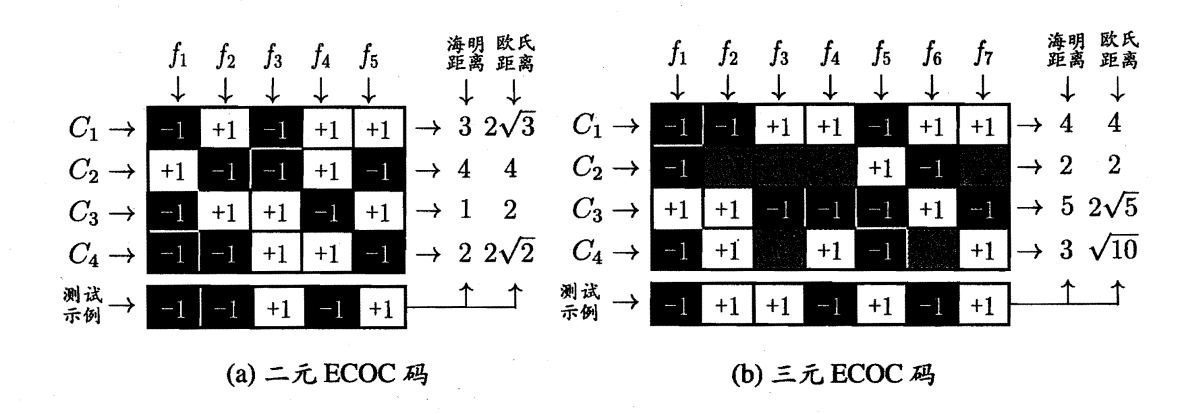# 机器学习（一）线性模型————理论篇 线性回归模型、对数几率模型、线性判别分析模型、多分类学习模型

## 线性模型基本介绍

$f(\boldsymbol{x})=\boldsymbol{w}^{\mathrm{T}} \boldsymbol{x}+b$

1. 基本线性回归模型
2. 对数几率回归模型
3. 线性判别分析模型
4. 多分类学习模型

## 基本线性回归模型

### 单元线性回归

• 建立含参线性模型

$f\left(x_i\right)=w x_i+b \text {, 使得 } f\left(x_i\right) \simeq y_i \text {. }$

• 构造性能度量

\begin{aligned} \left(w^*, b^*\right) & =\underset{(w, b)}{\arg \min } \sum_{i=1}^m\left(f\left(x_i\right)-y_i\right)^2 \\ & =\underset{(w, b)}{\arg \min } \sum_{i=1}^m\left(y_i-w x_i-b\right)^2 . \end{aligned}

• 参数估计

$w=\frac{\sum_{i=1}^m y_i\left(x_i-\bar{x}\right)}{\sum_{i=1}^m x_i^2-\frac{1}{m}\left(\sum_{i=1}^m x_i\right)^2}\\ b=\frac{1}{m} \sum_{i=1}^m\left(y_i-w x_i\right)$

### 多元线性回归

• 建立含参线性模型

$f\left(\boldsymbol{x}_i\right)=\boldsymbol{w}^{\mathrm{T}} \boldsymbol{x}_i+b, \text { 使得 } f\left(\boldsymbol{x}_i\right) \simeq y_i \text {, }$

• 构造性能度量

这里建立均方误差为性能度量：

$\hat{\boldsymbol{w}}^*=\underset{\hat{\boldsymbol{w}}}{\arg \min }(\boldsymbol{y}-\mathbf{X} \hat{\boldsymbol{w}})^{\mathrm{T}}(\boldsymbol{y}-\mathbf{X} \hat{\boldsymbol{w}})$

（1）为了便于讨论，这里将 $\boldsymbol{w}$$b$ 吸收合为一个向量 $\hat{\boldsymbol{w}} = (\boldsymbol{w};b)$ .相应的，把数据集表示为一个矩阵 $X$ ，其中每行对应一个样本，前 $d$ 列对应于每个样本的 $d$ 个属性值，最后一列元素恒为 1，即

$\mathbf{X}=\left(\begin{array}{ccccc} x_{11} & x_{12} & \ldots & x_{1 d} & 1 \\ x_{21} & x_{22} & \ldots & x_{2 d} & 1 \\ \vdots & \vdots & \ddots & \vdots & \vdots \\ x_{m 1} & x_{m 2} & \ldots & x_{m d} & 1 \end{array}\right)=\left(\begin{array}{cc} \boldsymbol{x}_1^{\mathrm{T}} & 1 \\ \boldsymbol{x}_2^{\mathrm{T}} & 1 \\ \vdots & \vdots \\ \boldsymbol{x}_m^{\mathrm{T}} & 1 \end{array}\right)$

（2）构造性能度量上式的推导

\begin{aligned} \hat{\boldsymbol{w}}^* & =\underset{\hat{\boldsymbol{w}}}{\arg \min } \sum_{i=1}^m\left(y_i-\hat{\boldsymbol{x}}_i^{\mathrm{T}} \hat{\boldsymbol{w}}\right)^2 \end{aligned}

$\hat{\boldsymbol{w}}^*=\underset{\hat{\boldsymbol{w}}}{\arg \min }\left[\begin{array}{lll} y_1-\hat{\boldsymbol{x}}_1^{\mathrm{T}} \hat{\boldsymbol{w}} & \cdots & y_m-\hat{\boldsymbol{x}}_m^{\mathrm{T}} \hat{\boldsymbol{w}} \end{array}\right]\left[\begin{array}{c} y_1-\hat{\boldsymbol{x}}_1^{\mathrm{T}} \hat{\boldsymbol{w}} \\ \vdots \\ y_m-\hat{\boldsymbol{x}}_m^{\mathrm{T}} \hat{\boldsymbol{w}} \end{array}\right]$

$\hat{\boldsymbol{w}}^*=\underset{\hat{\boldsymbol{w}}}{\arg \min }(\boldsymbol{y}-\mathbf{X} \hat{\boldsymbol{w}})^{\mathrm{T}}(\boldsymbol{y}-\mathbf{X} \hat{\boldsymbol{w}})$

• 参数估计

$\hat{\boldsymbol{w}}^*=\left(\mathbf{X}^{\mathrm{T}} \mathbf{X}\right)^{-1} \mathbf{X}^{\mathrm{T}} \boldsymbol{y}$

## 对数几率回归

### 广义线性模型介绍

$\ln y=\boldsymbol{w}^{\mathrm{T}} \boldsymbol{x}+b .$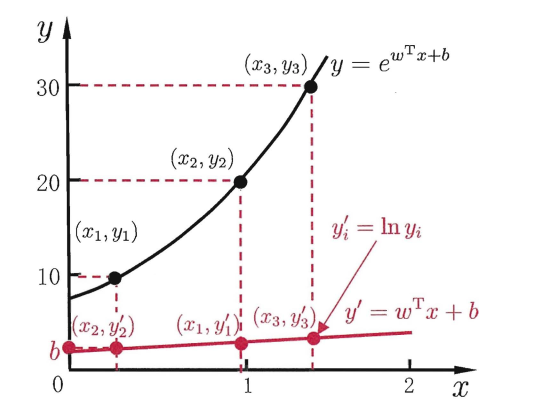$g(y) = \boldsymbol{w^Tx}+b$

### 对数几率回归模型

$g^{-1}(z)=\frac{1}{1+e^{-z}}$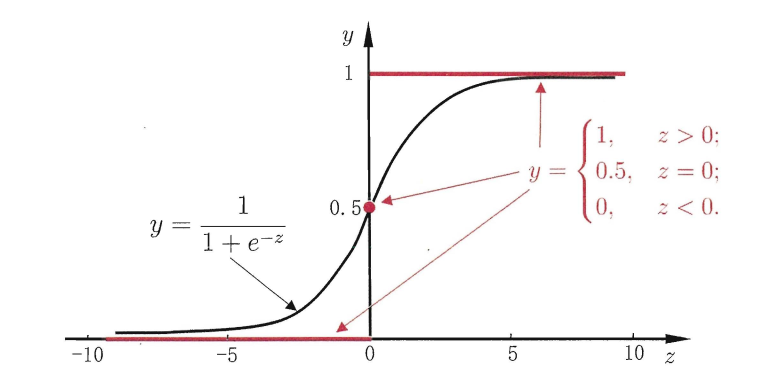• 建立含参线性模型

$y=\frac{1}{1+e^{\boldsymbol{w}^{\mathrm{T}} \boldsymbol{x}+b}} \\ \ln \frac{y}{1-y}=\boldsymbol{w}^{\mathrm{T}} \boldsymbol{x}+b$

$\ln \frac{y}{1-y}$

• 构造性能度量

$\ell(\boldsymbol{w}, b)=\sum_{i=1}^m \ln p\left(y_i \mid \boldsymbol{x}_i ; \boldsymbol{w}, b\right)$

$\ln \frac{p(y=1 \mid \boldsymbol{x})}{p(y=0 \mid \boldsymbol{x})}=\boldsymbol{w}^{\mathrm{T}} \boldsymbol{x}+b .$

\begin{aligned} & p(y=1 \mid \boldsymbol{x})=\frac{e^{\boldsymbol{w}^{\mathrm{T}} \boldsymbol{x}+b}}{1+e^{\boldsymbol{w}^{\mathrm{T}} \boldsymbol{x}+b}}, \\ & p(y=0 \mid \boldsymbol{x})=\frac{1}{1+e^{\boldsymbol{w}^{\mathrm{T}} \boldsymbol{x}+b}}, \end{aligned}

$\ell(\boldsymbol{\beta})=\sum_{i=1}^m\left(-y_i \boldsymbol{\beta}^{\mathrm{T}} \hat{\boldsymbol{x}}_i+\ln \left(1+e^{\boldsymbol{\beta}^{\mathrm{T}} \hat{\boldsymbol{x}}_i}\right)\right) .$

• 参数估计

$\boldsymbol{\beta}^*=\underset{\boldsymbol{\beta}}{\arg \min } \ell(\boldsymbol{\beta}) .$

## 线性判别分析

LDA 的思想为: 给定训练样例集, 设法将样例投影到一条直线上, 使得同类样例的投影点尽可能接近、不同类样例的投影点尽可能远离; 在对新样本进行分类时, 将其投影到同样的这条直线上, 再根据投影点的位置来确定新样本的类别. 如下图所示：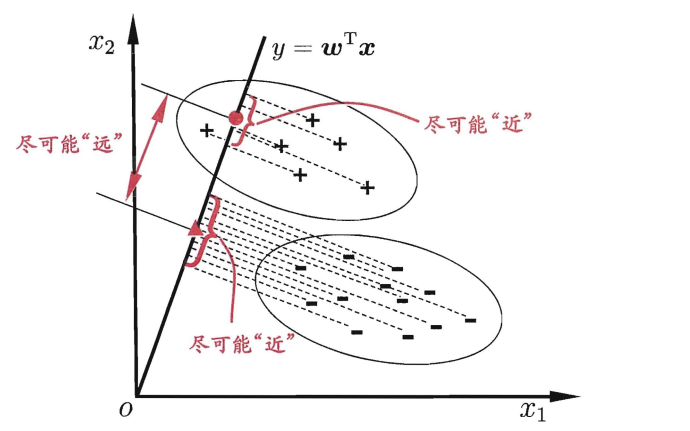### 建立模型

• $i$ 类示例的集合 $X_i$

• $i$ 类示例的均值向量 $\boldsymbol{\mu}_i$

• $i$ 类示例的协方差矩阵 $\boldsymbol{\Sigma}_i$

• 两类样本的中心在直线上的投影: $\boldsymbol{w}^{\mathrm{T}} \boldsymbol{\mu}_0$$\boldsymbol{w}^{\mathrm{T}} \boldsymbol{\mu}_1$

• 两类样本的协方差: $\boldsymbol{w}^{\mathrm{T}} \boldsymbol{\Sigma}_0 \boldsymbol{w}$$\boldsymbol{w}^{\mathrm{T}} \boldsymbol{\Sigma}_1 \boldsymbol{w}$

### 构造性能度量

$J=\frac{\left\|\boldsymbol{w}^{\mathrm{T}} \boldsymbol{\mu}_0-\boldsymbol{w}^{\mathrm{T}} \boldsymbol{\mu}_1\right\|_2^2}{\boldsymbol{w}^{\mathrm{T}} \boldsymbol{\Sigma}_0 \boldsymbol{w}+\boldsymbol{w}^{\mathrm{T}} \boldsymbol{\Sigma}_1 \boldsymbol{w}}=\frac{\boldsymbol{w}^{\mathrm{T}}\left(\boldsymbol{\mu}_0-\boldsymbol{\mu}_1\right)\left(\boldsymbol{\mu}_0-\boldsymbol{\mu}_1\right)^{\mathrm{T}} \boldsymbol{w}}{\boldsymbol{w}^{\mathrm{T}}\left(\boldsymbol{\Sigma}_0+\boldsymbol{\Sigma}_1\right) \boldsymbol{w}}$

### 参数估计

#### 补充 广义瑞利商

$R(A, x)=\frac{x^H A x}{x^H x}$

$\lambda_{\min } \leq \frac{x^H A x}{x^H x} \leq \lambda_{\max }$

$R(A, x)=\frac{x^H A x}{x^H B x}$

$R\left(A, B, x^{\prime}\right)=\frac{x^{\prime H} B^{-1 / 2} A B^{-1 / 2} x^{\prime}}{x^{\prime H} x^{\prime}}$

#### 参数估计的求解

$J=\frac{\left\|\boldsymbol{w}^{\mathrm{T}} \boldsymbol{\mu}_0-\boldsymbol{w}^{\mathrm{T}} \boldsymbol{\mu}_1\right\|_2^2}{\boldsymbol{w}^{\mathrm{T}} \boldsymbol{\Sigma}_0 \boldsymbol{w}+\boldsymbol{w}^{\mathrm{T}} \boldsymbol{\Sigma}_1 \boldsymbol{w}}=\frac{\boldsymbol{w}^{\mathrm{T}}\left(\boldsymbol{\mu}_0-\boldsymbol{\mu}_1\right)\left(\boldsymbol{\mu}_0-\boldsymbol{\mu}_1\right)^{\mathrm{T}} \boldsymbol{w}}{\boldsymbol{w}^{\mathrm{T}}\left(\boldsymbol{\Sigma}_0+\boldsymbol{\Sigma}_1\right) \boldsymbol{w}}$

\begin{aligned} \mathbf{S}_w & =\boldsymbol{\Sigma}_0+\boldsymbol{\Sigma}_1 \\ & =\sum_{\boldsymbol{x} \in X_0}\left(\boldsymbol{x}-\boldsymbol{\mu}_0\right)\left(\boldsymbol{x}-\boldsymbol{\mu}_0\right)^{\mathrm{T}}+\sum_{\boldsymbol{x} \in X_1}\left(\boldsymbol{x}-\boldsymbol{\mu}_1\right)\left(\boldsymbol{x}-\boldsymbol{\mu}_1\right)^{\mathrm{T}} \end{aligned}

$\mathbf{S}_b=\left(\boldsymbol{\mu}_0-\boldsymbol{\mu}_1\right)\left(\boldsymbol{\mu}_0-\boldsymbol{\mu}_1\right)^{\mathrm{T}},$

$J=\frac{\boldsymbol{w}^{\mathrm{T}} \mathbf{S}_b \boldsymbol{w}}{\boldsymbol{w}^{\mathrm{T}} \mathbf{S}_w \boldsymbol{w}}$

$\mathbf{S}_b \boldsymbol{w}=\lambda \mathbf{S}_w \boldsymbol{w}$

$\boldsymbol{w}=\mathbf{S}_w^{-1}\left(\boldsymbol{\mu}_0-\boldsymbol{\mu}_1\right)$

## 多分类学习问题

• 一对一 （OvO）
• 一对其余 （OvR）
• 多对多 （MvM）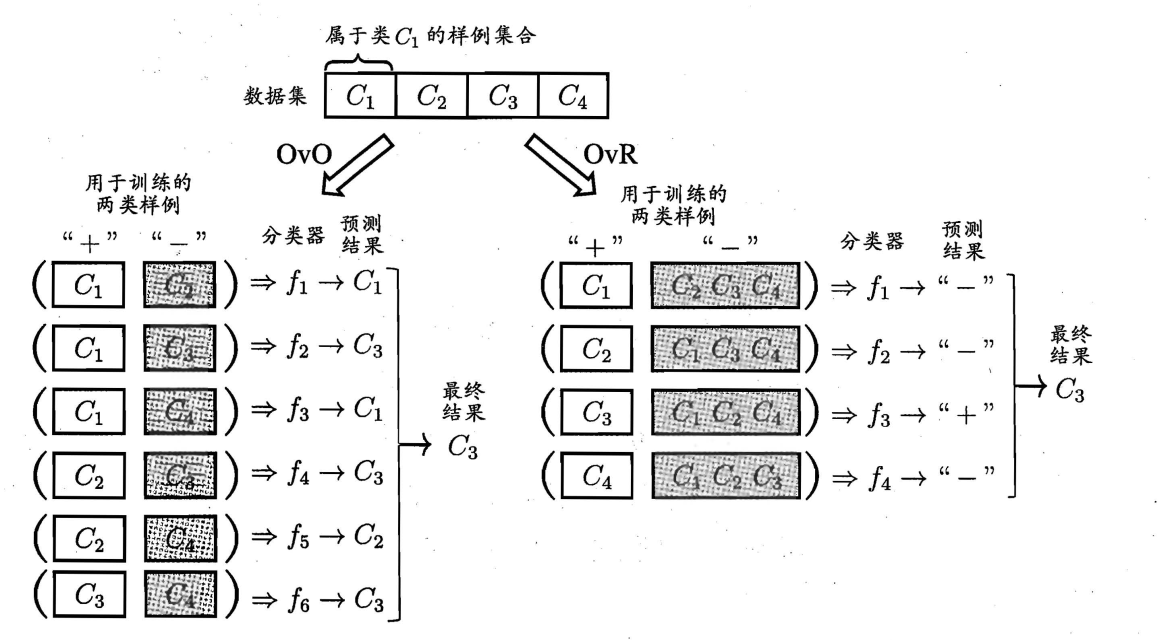$\mathrm{MvM}$ 是每次将若干个类作为正类, 若干个其他类作为反类. 显然, $\mathrm{OvO}$ 和 OvR 是 MvM 的特例. MvM 的正、反类构造必须有特殊的设计, 不能随意选取. 这里我们介绍一种最常用的 MvM 技术: “纠错输出码” (Error Correcting Output Codes, 简称 ECOC).

ECOC 是将编码的思想引入类别拆分, 并尽 可能在解码过程中具有容错性. ECOC 工作过程主要分为两步:

• 编码: 对 $N$ 个类别做 $M$ 次划分, 每次划分将一部分类别划为正类, 一部 分划为反类, 从而形成一个二分类训练集; 这样一共产生 $M$ 个训练集, 可 训练出 $M$ 个分类器.
• 解码: $M$ 个分类器分别对测试样本进行预测, 这些预测标记组成一个编 码. 将这个预测编码与每个类别各自的编码进行比较, 返回其中距离最小 的类别作为最终预测结果.